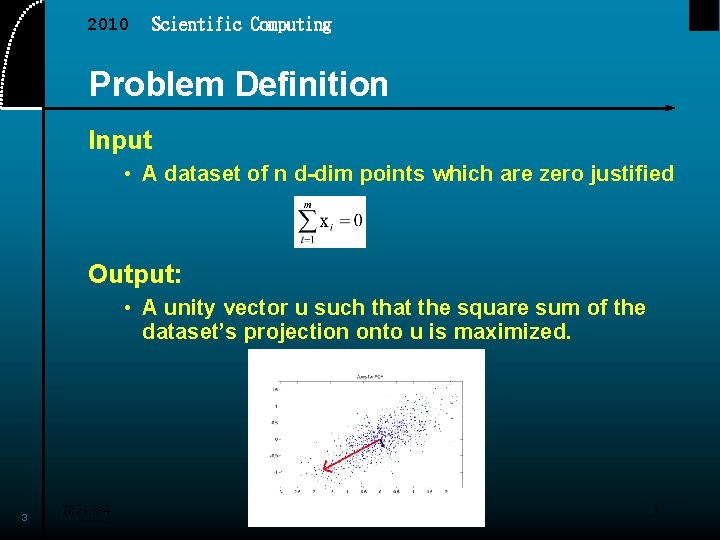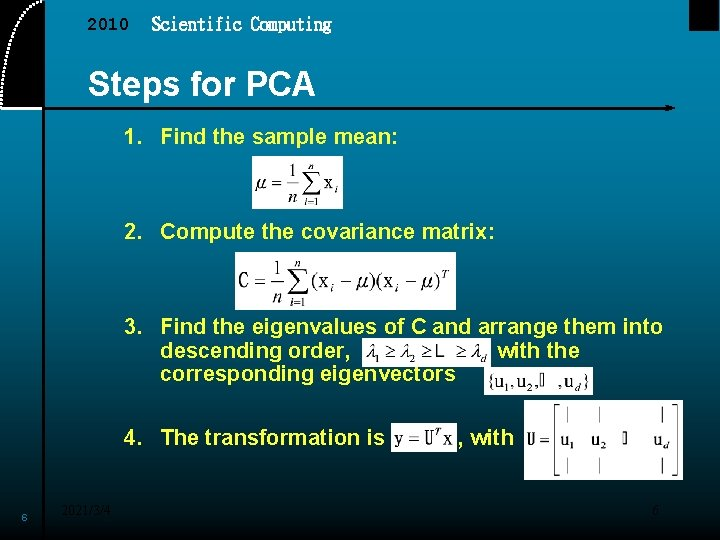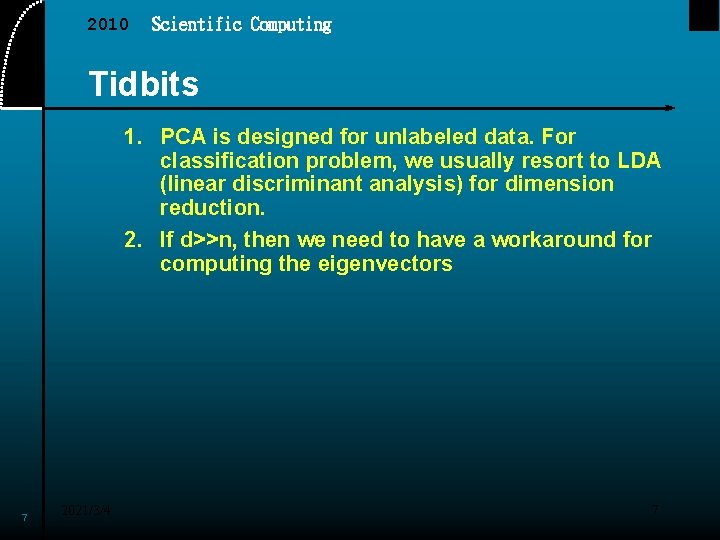# 2010 Scientific Computing Introduction PCA Principal Component Analysis

• Slides: 92010 Scientific Computing Introduction PCA (Principal Component Analysis) • An effective statistical method for reducing dataset dimensions while keeping spatial characteristics as much as possible • Applications - Image compression - Pattern recognition Characteristics: • Designed for unlabeled data • A linear transformation with solid mathematical foundation • Easy to apply 2 2021/3/4 22010 Scientific Computing Problem Definition Input • A dataset of n d-dim points which are zero justified Output: • A unity vector u such that the square sum of the dataset’s projection onto u is maximized. 3 2021/3/4 32010 Scientific Computing Math Formulation • Dataset representation: - X is d by n, with n>d • Projection of each column of X onto u: • Square sum: • Objective function with a constraint on u: 4 2021/3/4 42010 Scientific Computing Optimization of the Obj. Function Optimization • Set the gradient to zero: • Implication: - u is the eigenvector while l is the eigenvalue • When u is the eigenvector: 5 2021/3/4 • If we arrange eigenvalues such that: - Max of J(u) is l 1, which occurs at u=u 1 - Min of J(u) is ld, which occurs at u=ud 52010 Scientific Computing Steps for PCA 1. Find the sample mean: 2. Compute the covariance matrix: 3. Find the eigenvalues of C and arrange them into descending order, with the corresponding eigenvectors 4. The transformation is 6 2021/3/4 , with 62010 Scientific Computing Tidbits 1. PCA is designed for unlabeled data. For classification problem, we usually resort to LDA (linear discriminant analysis) for dimension reduction. 2. If d>>n, then we need to have a workaround for computing the eigenvectors 7 2021/3/4 72010 Scientific Computing Example of PCA IRIS dataset projection 8 2021/3/4 82010 Scientific Computing Weakness of PCA Not designed for classification problem (with labeled training data. Ideal situation 9 2021/3/4 Adversary situation 92010 Scientific Computing Linear Discriminant Analysis LDA projection onto directions that can best separate data of different classes. Adversary situation for PCA 10 2021/3/4 Ideal situation for LDA 10